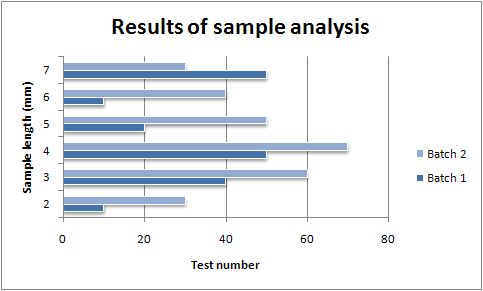and 1 contributors

# NAME

Bar - A class for writing Excel Bar charts.

# SYNOPSIS

To create a simple Excel file with a Bar chart using Excel::Writer::XLSX:

``````    #!/usr/bin/perl

use strict;
use warnings;
use Excel::Writer::XLSX;

my \$workbook  = Excel::Writer::XLSX->new( 'chart.xlsx' );
my \$worksheet = \$workbook->add_worksheet();

my \$chart     = \$workbook->add_chart( type => 'bar' );

# Configure the chart.
\$chart->add_series(
categories => '=Sheet1!\$A\$2:\$A\$7',
values     => '=Sheet1!\$B\$2:\$B\$7',
);

# Add the worksheet data the chart refers to.
my \$data = [
[ 'Category', 2, 3, 4, 5, 6, 7 ],
[ 'Value',    1, 4, 5, 2, 1, 5 ],
];

\$worksheet->write( 'A1', \$data );

__END__``````

# DESCRIPTION

This module implements Bar charts for Excel::Writer::XLSX. The chart object is created via the Workbook `add_chart()` method:

``    my \$chart = \$workbook->add_chart( type => 'bar' );``

Once the object is created it can be configured via the following methods that are common to all chart classes:

``````    \$chart->add_series();
\$chart->set_x_axis();
\$chart->set_y_axis();
\$chart->set_title();``````

These methods are explained in detail in Excel::Writer::XLSX::Chart. Class specific methods or settings, if any, are explained below.

# Bar Chart Subtypes

The `Bar` chart module also supports the following sub-types:

``````    stacked
percent_stacked``````

These can be specified at creation time via the `add_chart()` Worksheet method:

``    my \$chart = \$workbook->add_chart( type => 'bar', subtype => 'stacked' );``

# EXAMPLE

Here is a complete example that demonstrates most of the available features when creating a chart.

``````    #!/usr/bin/perl

use strict;
use warnings;
use Excel::Writer::XLSX;

my \$workbook  = Excel::Writer::XLSX->new( 'chart_bar.xlsx' );
my \$worksheet = \$workbook->add_worksheet();
my \$bold      = \$workbook->add_format( bold => 1 );

# Add the worksheet data that the charts will refer to.
my \$headings = [ 'Number', 'Batch 1', 'Batch 2' ];
my \$data = [
[ 2,  3,  4,  5,  6,  7 ],
[ 10, 40, 50, 20, 10, 50 ],
[ 30, 60, 70, 50, 40, 30 ],

];

\$worksheet->write( 'A1', \$headings, \$bold );
\$worksheet->write( 'A2', \$data );

# Create a new chart object. In this case an embedded chart.
my \$chart = \$workbook->add_chart( type => 'bar', embedded => 1 );

# Configure the first series.
\$chart->add_series(
name       => '=Sheet1!\$B\$1',
categories => '=Sheet1!\$A\$2:\$A\$7',
values     => '=Sheet1!\$B\$2:\$B\$7',
);

# Configure second series. Note alternative use of array ref to define
# ranges: [ \$sheetname, \$row_start, \$row_end, \$col_start, \$col_end ].
\$chart->add_series(
name       => '=Sheet1!\$C\$1',
categories => [ 'Sheet1', 1, 6, 0, 0 ],
values     => [ 'Sheet1', 1, 6, 2, 2 ],
);

# Add a chart title and some axis labels.
\$chart->set_title ( name => 'Results of sample analysis' );
\$chart->set_x_axis( name => 'Test number' );
\$chart->set_y_axis( name => 'Sample length (mm)' );

# Set an Excel chart style. Blue colors with white outline and shadow.
\$chart->set_style( 11 );

# Insert the chart into the worksheet (with an offset).
\$worksheet->insert_chart( 'D2', \$chart, 25, 10 );

__END__``````

This will produce a chart that looks like this:# AUTHOR

John McNamara jmcnamara@cpan.org

Copyright MM-MMXX, John McNamara.

All Rights Reserved. This module is free software. It may be used, redistributed and/or modified under the same terms as Perl itself.